###Calculating Feet and Inches (base 12)

The duodecimal or dozenal number system is a base 12 system. In this system, the number ten may be written as "A", "T", or "X", and the number eleven as "B" or "E".

In a recent post to the Soroban/Abacus newsgroup group member Hannu Hinkka suggested using an inverted suan pan as an interesting way to calculate in base 12. Taking Hannu?s ideas and those of group member Steve Treadwell brings me to this post. Thank you to them both.

In this tutorial I'll show the process for addition; simply reverse the procedure for subtraction. Before beginning it's important to be familiar with the tools needed to add and subtract in base 12. The first image below shows an inverted suan with each rod representing a base 12 numeral. Above the beam we use only 3 beads, each has a value of 3. Below the beam we use two beads, each has a value of 1.

#####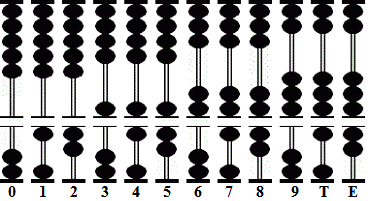Base 12 numerals on an inverted suan Pan

Those familiar with the addition and subtraction techniques in Abacus: Mystery of the Bead will know how much we rely on the complementary numbers for base 10. This second image shows the complementary numbers for base 12.

 1 2 3 4 5 6 5 4 3 2 1 E T 9 8 7 6 7 8 9 T E
##### Complementary Numbers for Base 12

Example 1: Add 0' 9? + 0' 9? + 0' 9? + 0' 9? = 3? 0?Step 1: Set 9 inches onto the abacus (rod J will act as the unit rod for feet, rod L for inches)Step 2: Add 9 to rod L. In order to do this consult the complementary number chart. Subtract the complementary 3 and add 1 to rod J. Interim answer is 1' 6".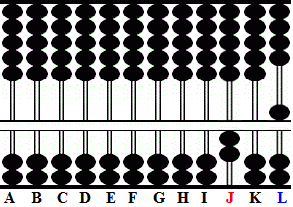Step 3: Add 9 to rod L. Once again subtract the complement 3 and add 1 to rod J. Interim answer is 2' 3".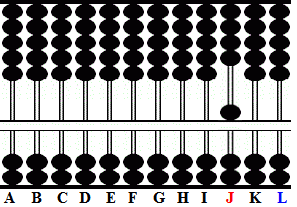Step 4 and the answer: Add 9 to rod L. Subtract the complementary 3 and add 1 to rod J leaving the answer 3' 0".

Example 2: Add 0' 11" + 0' 10" = 1' 9"

#### ? ? ? In base 12, numerals 10 and 11 are given symbols. When adding inches treat them as numbers. ? ? ?Step 1: Set 11 inches onto the abacus (rod J will act as the unit rod for feet, rod  L for inches)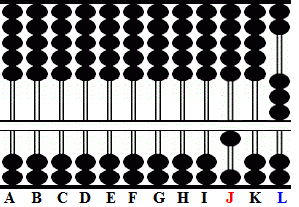Step 2 and the answer: Add 10 to rod L. Subtract the complementary 2 and add 1 to rod J leaving the answer 1' 9".

Example 3: Add 9' 9" + 3' 7" = 13' 4"

#### ? ? ? This example requires complementary number operations in both base 10 and for base 12. ? ? ?Step 1: Set 9' 9" onto the abacus (rod J will act as the unit rod for feet, rod  L for inches)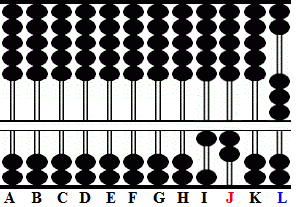This is where some care is needed. When adding numbers to the feet portion of the equation we are not working in base 12. Instead use the complementary numbers for base 10.Step 2: Add 3' to 9' on rod J. Subtract the complementary 7 from 9 and carry 1 to the left leaving the interim answer 12' 9".Step 3 and the answer: Add 7 to rod L. Subtract the complementary 5 and add 1 to rod J leaving the answer 13' 4".